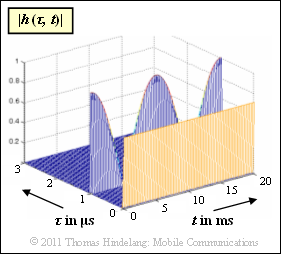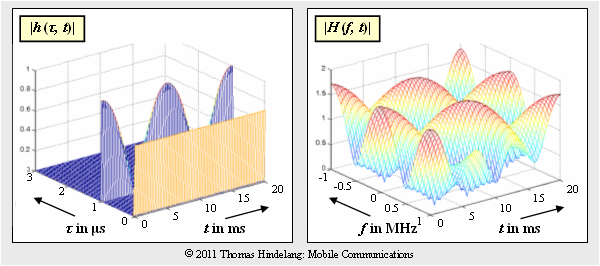# Exercise 2.4: 2D Transfer Function2D impulse response  $|h(\tau, \hspace{0.05cm}t)|$

The graph shows the two-dimensional impulse response  $h(\tau, \hspace{0.05cm}t)$  of a mobile radio system in magnitude representation.

• It can be seen that the 2D impulse response only has components at delays  $\tau = 0$  and  $\tau = 1 \ \rm µ s$ .
• At these times:
$$h(\tau = 0\,{\rm µ s},\hspace{0.05cm}t) \hspace{-0.1cm} \ = \ \hspace{-0.1cm} \frac{1}{ \sqrt{2}} = {\rm const.}$$
$$h(\tau = 1\,{\rm µ s},\hspace{0.05cm}t) \hspace{-0.1cm} \ = \ \hspace{-0.1cm} \cos(2\pi \cdot {t}/{ T_0})\hspace{0.05cm}.$$

For all other values of  $\tau$,  we have  $h(\tau, \hspace{0.05cm}t) \equiv 0$.

We want to obtain the two-dimensional transfer function  $H(f, \hspace{0.05cm} t)$  as the Fourier transform of  $h(\tau, t)$  with respect to the delay   $\tau$:

$$H(f,\hspace{0.05cm} t) \hspace{0.2cm} \stackrel {f,\hspace{0.05cm}\tau}{\bullet\!\!-\!\!\!-\!\!\!-\!\!\circ} \hspace{0.2cm} h(\tau,\hspace{0.05cm}t) \hspace{0.05cm}.$$

Notes:

### Questionnaire

1

What is the period   $T_0$  of the function  $h(\tau = 1 \ {\rm µ s},\hspace{0.05cm} t)$?  Note that the graph shows the magnitude  $|h(\tau, \hspace{0.05cm}t)|$ .

 $T_0 \ = \$ $\ \ \rm ms$

2

At what times  $t_1$  $($between  $0$  and  $10 \ \rm ms)$  and  $t_2$  $($between  $10 \ \ \rm ms$  and  $20 \ \ \rm ms)$  is  $H(f, \hspace{0.05cm}t)$  constant in $f$  ?

 $t_1 \ = \$ $\ \ \rm ms$ $t_2 \ = \$ $\ \ \rm ms$

3

Calculate  $H_0(f) = H(f, \hspace{0.05cm}t = 0)$.  Which statements are true?

 We have  $H_0(f) = H_0(f + i \cdot 1 \ {\rm MHz}), \ i = ±1, ±2, \ \ \text{...}$ We have approximately  $0.293 ≤ |H_0(f)| ≤ 1.707$. $|H_0(f)|$  has a maximum at $f = 0$ .

4

Calculate  $H_{10}(f) = H(f, \hspace{0.05cm}t = 10 \ \rm ms)$.  Which statements are true?

 We have  $H_{10}(f) = H_{10}(f + i \cdot 1 \ {\rm MHz}),\ i = ±1, ±2, \ \ \text{...}$ We have approximately  $0.293 ≤ H_{10}(f) ≤ 1.707$. $|H_{10}(f)|$  has a maximum at $f = 0$ .

### Solution

#### Solution

(1)  The period can be read from the given graph.  If the magnitude representation is taken into account, the result is  $T_0 \ \underline {= 20 \ \ \rm ms}$.

(2)  At time  $t_1 \ \underline {= 5 \ \ \rm ms}$  we have  $h(\tau = 1 \ {\rm µ s}, t_1) = 0$.  Accordingly, the following applies

$$h(\tau = 1\,{\rm µ s},\hspace{0.05cm}t_1) = \frac{1}{ \sqrt{2}} \cdot \delta(\tau)\hspace{0.3cm}\Rightarrow \hspace{0.3cm} H(f,\hspace{0.05cm}t_1) = \frac{1}{ \sqrt{2}} = {\rm const.}$$

The same applies to  $t_2 \ \underline {= 15 \ \ \rm ms}$:

$$h(\tau = 1\,{\rm µ s},\hspace{0.05cm}t_2) = \frac{1}{ \sqrt{2}} \cdot \delta(\tau)\hspace{0.3cm}\Rightarrow \hspace{0.3cm} H(f,\hspace{0.05cm}t_2) = \frac{1}{ \sqrt{2}} = {\rm const.}$$

(3)  At time  $t = 0$  the impulse response with  $\tau_1 = 1 \ \ \rm µ s$  is

$$h(\tau,\hspace{0.05cm}t = 0) = \frac{1}{ \sqrt{2}} \cdot \delta(\tau)+ \delta(\tau - \tau_1)\hspace{0.05cm}.$$

Its Fourier transform is

$$H_0(f) = H(f,\hspace{0.05cm}t = 0) \hspace{-0.1cm} \ = \ \hspace{-0.1cm} \frac{1}{ \sqrt{2}} + 1 \cdot {\rm e}^{- {\rm j}\cdot 2 \pi f \tau_1}=\frac{1}{ \sqrt{2}} + \cos( 2 \pi f \tau_1)- {\rm j}\cdot \sin( 2 \pi f \tau_1)$$
$$\Rightarrow \hspace{0.3cm} |H_0(f)| \hspace{-0.1cm} \ = \ \hspace{-0.1cm} \sqrt { \left [ {1}/{ \sqrt{2}} + \cos( 2 \pi f \tau_1) \right ]^2 + \left [\sin( 2 \pi f \tau_1)\right ]^2}= \sqrt { 0.5 + 1 + {2}/{ \sqrt{2}} \cdot \cos( 2 \pi f \tau_1)} = \sqrt { 1.5 + { \sqrt{2}} \cdot \cos( 2 \pi f \tau_1)}\hspace{0.05cm}.$$

Therefore,

• $H_0(f)$  is periodic with  $1/\tau_1 = 1 \ \rm MHz$.
• For the maximum and minimum values, we have
$${\rm Max}\, \left [ \, |H_0(f)|\, \right ] \hspace{-0.1cm} \ = \ \hspace{-0.1cm} \sqrt { 1.5 + { \sqrt{2}} } \approx 1.707 \hspace{0.05cm},\hspace{0.5cm}{\rm Min}\, \left [ \, |H_0(f)|\, \right ] \hspace{-0.1cm} \ = \ \hspace{-0.1cm} \sqrt { 1.5 - { \sqrt{2}} } \approx 0.293 \hspace{0.05cm}.$$
• At $f = 0$,  $|H_0(f)|$ has a maximum.

Therefore, all three solution suggestions  are correct.

(4)  For the time $t = 10 \ \rm ms$ the following equations apply:

$$h(\tau,\hspace{0.05cm}t = 10\,{\rm ms}) \hspace{-0.1cm} \ = \ \hspace{-0.1cm} \frac{1}{ \sqrt{2}} \cdot \delta(\tau)- \delta(\tau - \tau_1)\hspace{0.05cm},$$
$$H_{10}(f) = H(f,\hspace{0.05cm}t = 10\,{\rm ms}) \hspace{-0.1cm} \ = \ \hspace{-0.1cm} \frac{1}{ \sqrt{2}} - \cos( 2 \pi f \tau_1)+ {\rm j}\cdot \sin( 2 \pi f \tau_1)\hspace{0.05cm},$$
$$|H_{10}(f)| \hspace{-0.1cm} \ = \ \hspace{-0.1cm} \sqrt { 1.5 - { \sqrt{2}} \cdot \cos( 2 \pi f \tau_1)}\hspace{0.05cm}.$$2D impulse response $|h(\tau, \hspace{0.05cm}t)|$ and 2D transfer function $|H(f, \hspace{0.05cm}t)|$

Solutions 1 and 2 are correct:

• The frequency period does not change compaired to  $t = 0$.
• The maximum value is still  $1.707$  and the minimum value  $0.293$  does not change compared to the subtask  (3).
• For $f = 0$  there is now a minimum and not a maximum.

The graph on the right shows the magnitude  $|H(f, t)|$  of the 2D transfer function.